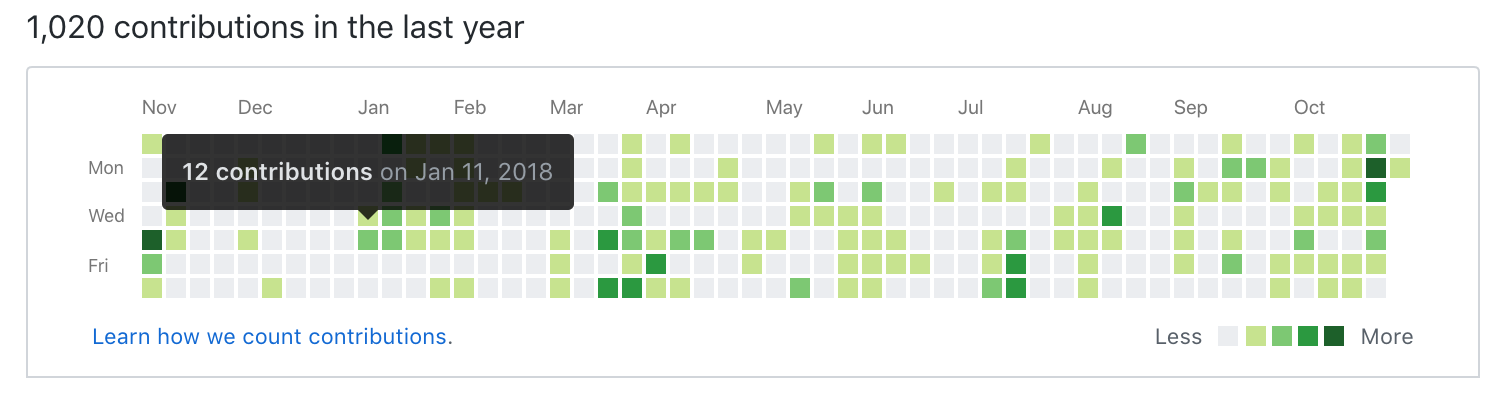### Introduction

Time series is a series of data that is indexed in time order. The time order can be expressed as day, week, month or year. The most common way to visualize time series data is to use a simple line chart, where the horizontal axis plots the increments of time and the vertical axis plots the variable that is being measured. The visualization can be achieved using `geom_line()` in `ggplot2` or simply using the `plot()` function in `Base R`.

In this tutorial, I will introduce a new tool to visualize Time Series Data called Time-Series Calendar Heatmap. We will look at how Time-Series Calendar Heatmaps can be drawn using `ggplot2`. We will also explore the `calendarHeat()` function written by Paul Bleicher (released as open source under GPL license) which provides an easy way to create the visualization.

### Motivation

If you have ever been to GitHub, you would have definitely stumpled across the below chart which shows the number of contrubtions made by a user over the past year. The color of the tiles are representative of the number of contributions (as described by the legend at the bottom right of the chart). Here, the calendar heatmap provides a visually appealing way to visualize over days in a calendar-like view, making it easy to identify daily patterns or anomalies.Another great example can be found on the below article by Wall Street Journal, which shows the number of infected people measured over 70 years across all 50 states in the US.

http://graphics.wsj.com/infectious-diseases-and-vaccines/

Over here, the calendar heatmap makes it easy to identify yearly patterns in the number of infected people for various diseases.

### Case Study

To illustrate the use of Heatmap Calendars, we will visualize Amazon’s stock price (NASDAQ: AMZN) over the past 5 years. We will be looking at the Adjusted Closing Prices, which will be obtained through the `tidyquant’ package.

### Packages

We will install and import the `tidyquant` package to obtain the stock prices of Amazon. We will also install and import `ggplot2` as to perform the visualization. The R code for `calendarHeat()` can be downloaded through Paul Bleicher’s Github page.

``````# install tidyquant
#install.packages('tidyquant', repos = "http://cran.us.r-project.org")
library(tidyquant)

#install ggplot2
#install.packages("ggplot2", repos = "http://cran.us.r-project.org")
library(ggplot2)

#Load the function to the local through Paul Bleicher's GitHub page
source("https://raw.githubusercontent.com/iascchen/VisHealth/master/R/calendarHeat.R")``````

``````amznStock = as.data.frame(tidyquant::tq_get(c("AMZN"),get="stock.prices")) # get data using tidyquant
amznStock = amznStock[year(amznStock\$date) > 2012, ] # Using data only after 2012``````

### Using `ggplot2`

The process of creating a calendar heatmap with ggplot2 is somewhat cumbersome. We need to get the data in the right shape before the heatmap can be plotted. The below code lists the step as to how we can munge the data for creating the calendar heatmap using `ggplot2`.

``````library(plyr)
library(plotly)

amznStock\$weekday = as.POSIXlt(amznStock\$date)\$wday #finding the day no. of the week
amznStock\$weekdayf<-factor(amznStock\$weekday,levels=rev(1:7),labels=rev(c("Mon","Tue","Wed","Thu","Fri","Sat","Sun")),ordered=TRUE) # converting the day no. to factor

amznStock\$monthf<-factor(month(amznStock\$date),levels=as.character(1:12),labels=c("Jan","Feb","Mar","Apr","May","Jun","Jul","Aug","Sep","Oct","Nov","Dec"),ordered=TRUE) # finding the month

amznStock\$yearmonth<- factor(as.yearmon(amznStock\$date)) # finding the year and the month from the date. Eg: Nov 2018

amznStock\$week <- as.numeric(format(amznStock\$date,"%W")) # finding the week of the year for each date

amznStock<-ddply(amznStock,.(yearmonth),transform,monthweek=1+week-min(week)) # normalizing the week to start at 1 for every month

p <- ggplot(amznStock, aes(monthweek, weekdayf, fill = amznStock\$adjusted)) +
geom_tile(colour = "white") + facet_grid(year(amznStock\$date)~monthf) + scale_fill_gradient(low="red", high="green") +  xlab("Week of Month") + ylab("") + ggtitle("Time-Series Calendar Heatmap: AMZN Stock Prices") + labs(fill = "Price")

p``````